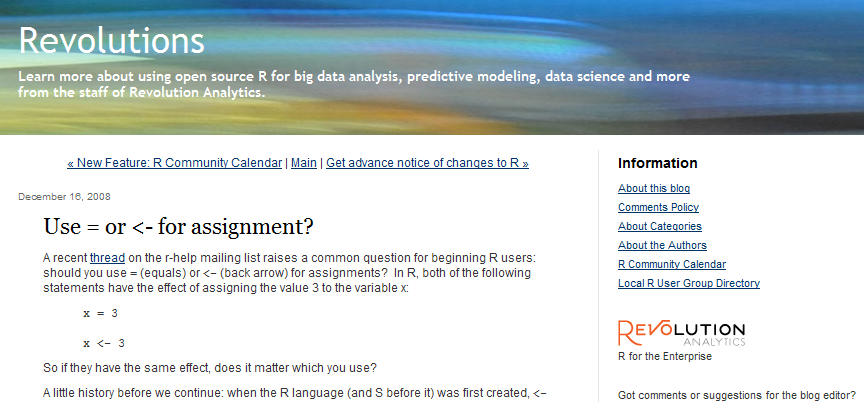# Recommended: Use = or <- for assignment?

I learned R back when the only way to assign a value was with the <- operator. For example, if you were computing the hypotenuse of a triangle, you’d use c <- sqrt(a^2+b^2). But the language now allows you to use an equals sign instead, which is the choice in many other programming languages. Should you do this? I say “no” emphatically, but this website makes an interesting counter-argument.

David Smith, Use = or <- for assignment. Revolutions Blog, December 16, 2008. Excerpt: “A recent thread on the r-help mailing list raises a common question for beginning R users: should you use = (equals) or <- (back arrow) for assignments? In R, both of the following statements have the effect of assigning the value 3 to the variable x: x = 3 or x <- 3. So if they have the same effect, does it matter which you use?” Available at http://blog.revolutionanalytics.com/2008/12/use-equals-or-arrow-for-assignment.html.